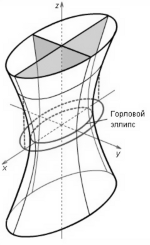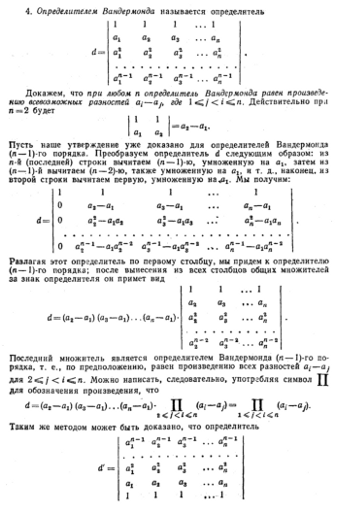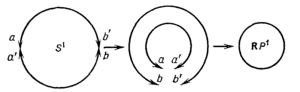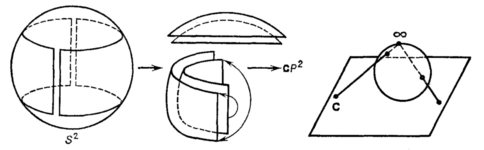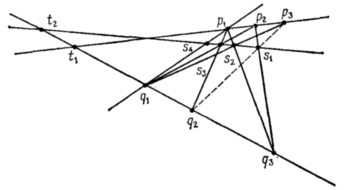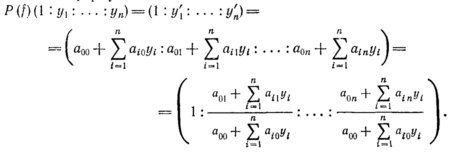# Peter Kogut's Books

#### 2020

1. Peter Kogut, Olha Kupenko, Approximation Methods in Optimization of Nonlinear Systems, De Gruyter Series in Nonlinear Analysis and Applications 32, Walter de Gruyter GmbH, Berlin/Boston, 2020, 350p.

A monograph on the subject Optimal Control of Strongly Nonlinear Partial Differential Equations is intended to address some of the obstacles that face researchers today, particularly with regard to ill-posedness of boundary value problems and problems where we can not expect to have uniqueness of their solutions in the standard functional spaces. Bringing original results together with others previously scattered across the literature, it tackles computational challenges by exploiting methods of approximation and asymptotic analysis and harnessing differences between optimal control problems and their underlying PDEs. The book is focused on self-contained development and study of the asymptotic and approximation methods of optimal control problems for strongly nonlinear elliptic systems which are related to many fields of applied mathematics, including combustion theory, thermal inflammation in chemical reactors, material sciences, mechanics of fluids, nonlinear dielectric composites, material and topology optimization. Specific topics covered in the work include: - a mostly self-contained mathematical theory of optimal control problems of elliptic equations with exponential type of nonlinearity and Dirichlet-Neumann boundary conditions; - a wide class of approximation optimal control problems which can be considered as a basis for the construction of suboptimal controls for the original control problems; - optimal control problems dealing with ill-posed objects such as elliptic equations with degenerate and unbounded coefficients in the principle part, variational inequalities with anisotropic \$p\$-Laplacian, elliptic equations with \$\mbox{BMO}\$-coefficients and \$L^1\$-right hand side; - asymptotic analysis of optimal control problems for nonlinear systems in domains with rugous boundary; - optimal and quasi-optimal control in coefficients for multi-dimensional thermistor problem; - the concept of consistent optimal control problems for ill-posed boundary value problems; - optimal control problems in coefficients for elliptic systems with variable \$p(x)\$-Laplacian. Served as a monograph where specific applications are explored, this book can be considered as a good reference for graduate students in pure and applied mathematics, as well as engineers, researchers, and practitioners requiring a solid mathematical basis for the solution of practical problems.

#### 2011

1. P. I. Kogut, G. Leugering, Optimal Control Problems for Partial Differential Equations on Reticulated Domains: Approximation and Asymptotic Analysis, Birkhäuser, Boston, 2011. 636p.

Introduction.- Part I. Asymptotic Analysis of Constrained Optimal Control Problems for Partial Differential Equations: Basic Tools.- Background Material on Asymptotic Analysis of External Problems.- Variational Methods of Optimal Control Theory.- Suboptimal and Approximate Solutions to External Problems.- Introduction to the Asymptotic Analysis of Optimal Control Problems: A Parade of Examples.- Convergence Concepts in Variable Banach Spaces.- Convergence of Sets in Variable Spaces.- Passing to the Limit in Constrained Minimization Problems.- Part II. Optimal Control Problems on Periodic Reticulated Domains: Asymptotic Analysis and Approximate Solutions.- Suboptimal Control of Linear Steady-States Processes on Thin Periodic Structures with Mixed Boundary Controls.- Approximate Solutions of Optimal Control Problems for Ill-Posed Objects on Thin Periodic Structures.- Asymptotic Analysis of Optimal Control Problems on Periodic Singular Structures.- Suboptimal Boundary Control of Elliptic Equations in Domains with Small Holes.- Asymptotic Analysis of Elliptic Optimal Control Problems in Thick Multi-Structures with Dirchlet and Neumann Boundary Controls.- Gap Phenomenon in Modeling of Suboptimal Controls to Parabolic Optimal Control Problems in Thick Multi-Structures.- Boundary Velocity Suboptimal Control of Incompressible Flow in Cylindrically Perforated Domains.- Optimal Control Problems in Coefficients: Sensitivity Analysis and Approximation.- References.- Index.
After over 50 years of increasing scientific interest, optimal control of partial differential equations (PDEs) has developed into a well-established discipline in mathematics with myriad applications to science and engineering. As the field has grown, so too has the complexity of the systems it describes; the numerical realization of optimal controls has become increasingly difficult, demanding ever more sophisticated mathematical tools.
A comprehensive monograph on the subject, Optimal Control of Partial Differential Equations on Reticulated Domains is intended to address some of the obstacles that face researchers today, particularly with regard to multi-scale engineering applications involving hierarchies of grid-like domains. Bringing original results together with others previously scattered across the literature, it tackles computational challenges by exploiting asymptotic analysis and harnessing differences between optimal control problems and their underlying PDEs. The book consists of two parts, the first of which can be viewed as a compendium of modern optimal control theory in Banach spaces. The second part is a focused, in-depth, and self-contained study of the asymptotics of optimal control problems related to reticulated domains-the first such study in the literature. Specific topics covered in the work include:
* a mostly self-contained mathematical theory of PDEs on reticulated domains;
* the concept of optimal control problems for PDEs in varying such domains, and hence, in varying Banach spaces;
* convergence of optimal control problems in variable spaces;
* an introduction to the asymptotic analysis of optimal control problems;
* optimal control problems dealing with ill-posed objects on thin periodic structures, thick periodic singular graphs, thick multi-structures with Dirichlet and Neumann boundary controls, and coefficients on reticulated structures.
Serving as both a text on abstract optimal control problems and a monograph where specific applications are explored, this book can be used as reference for graduate students, researchers, and practitioners in mathematics and areas of engineering involving reticulated domains. (see http://www.springer.com/alert/urltracking.do?id=L1d9d8bMc8aa74Saf4bd9b)

#### 2010

1. О. П. Когут, П. І. Когут, О. А. Рядно, Оптимізація в нелінійних еліптичних крайових задачах, ДДФА, Дніпропетровськ, 2010. 236 c.

Монографія присвячена дослідженню задач оптимального керування в коефіцієнтах систем, які описуються лінійними та нелінійними еліптичними рівняннями. В якості допустимих керувань в коефіцієнтах головної частини нелінійних еліптичних операторів запропоновано ввести новий клас недиференційовних функцій, що забезпечує розв'язність відповідних оптимізаційних задач. Також у роботі розглядаються задачі оптимального керування в коефіцієнтах для вироджених еліптичних систем. Такі задачі можуть набувати різних постановок, що залежать від вибору вагових функціональних просторів.
Монографія розрахована на студентів та аспірантів природничих факультетів, а також спеціалістів з проблем оптимального керування та оптимізації.